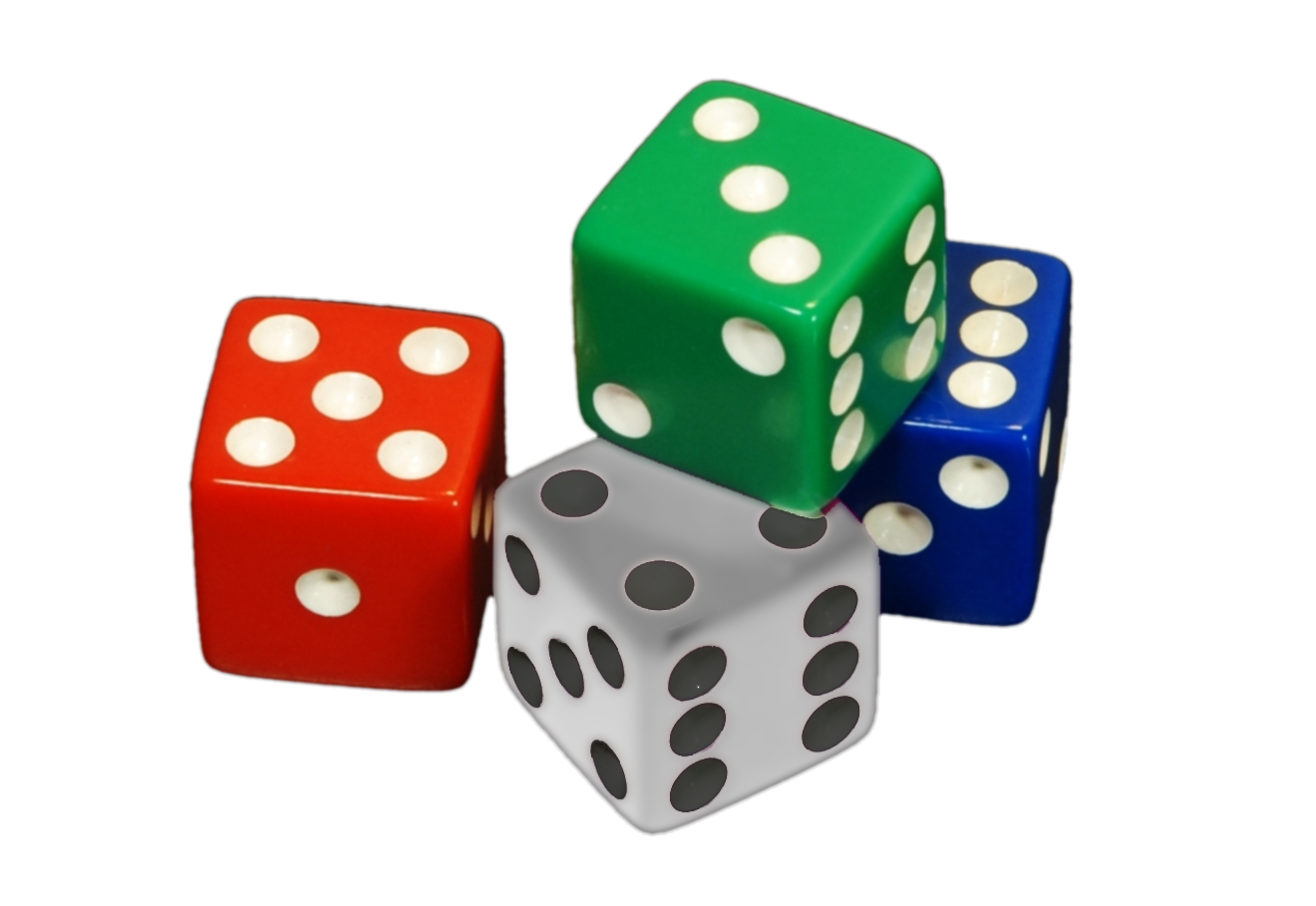## ________________

In this year’s Math League Summer Tournament for middle-school students from Asia and North America, held at the College of New Jersey, participants studied the math of dice.

## ________________### Two Sums

Deena has a white die, a red die, a green die, and a blue die. All are ordinary, fair six-sided dice. If she rolls all four of them, there are 56 different outcomes in which the sum of the pips showing on top is nine.

Only one other number occurs as the sum of the top faces in exactly 56 outcomes. What is it?

### Double-Faced

Charles borrows one of Deena’s dice and rolls it six times.

What is the probability that at least one of the numbers one through six occurs more than once in those six rolls?

## Solutions to week 102

Zombie Epidemic. First notice that the size of every zombie colony is a power of two (the exponents being 1, 1, 2, 3, 4, 5, 6, and 7) and that when two colonies combine, their sizes are multiplied and then divided by two. That result will also be a power of two; in fact, the operation corresponds to adding the exponents and subtracting one from the result to get the exponent of two in the answer. Also notice that this operation is both commutative and associative — in other words, it doesn’t matter in what order the colonies combine. The final number of zombies will always be the same: a power of two with exponent equal to the sum of all of the original exponents, minus one for each of the merges that had to take place to get down to a single colony. Since there are eight colonies to begin with, it takes seven merges to get down to one colony. Hence, the final exponent of two will be 1+1+2+3+4+5+6+7-7 = 22, meaning that there will be 222 = 4,194,304 zombies.

Revenge on the Zombies. Since every group of zombies has either four or five members, the total number of zombies left is somewhere between 19×4 = 76 and 19×5 = 95, inclusive. But there is only one multiple of 17 in this range, namely 85. So we know there are exactly 85 zombies, which we can only arrive at with ten groups of four and nine groups of five, since 4×10 + 5×9 = 85.

Suppose you change the number of groups of zombies from 19 to some other value, but change nothing else about the problem. For which numbers of groups will the puzzle have a unique solution?

## Recent Weeks

Links to all of the puzzles and solutions are on the Complete Varsity Math page.

Come back next week for answers and more puzzles.